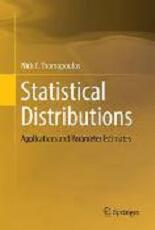SFr. 93.00 € 93.00BTC 0.0053LTC 1.212ETH 0.1692bestellen Artikel-Nr. 27602842 Diesen Artikel in meineWunschliste Diesen Artikel weiterempfehlen Diesen Preis beobachten Weitersagen: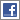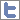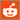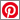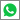Autor(en): Nick T. Thomopoulos
 Statistical Distributions: Applications and Parameter Estimates(Buch)
Dieser Artikel gilt, aufgrund seiner Grösse, beim Versand als 2 Artikel!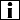Übersicht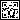Auf mobile öffnenLieferstatus: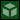i.d.R. innert 5-10 Tagen versandfertig Genre: Schulbücher ISBN: 9783319879529 EAN-Code: 9783319879529 Verlag: Springer International Publishing Einband: Kartoniert Sprache: English Dimensionen: H 236 mm / B 156 mm / D 15 mm Gewicht: 306 gr Seiten: 192 Zus. Info: Paperback Bewertung: Titel bewerten / Meinung schreiben
 Inhalt: This book gives a description of the group of statistical distributions that have ample application to studies in statistics and probability. Understanding statistical distributions is fundamental for researchers in almost all disciplines.  The informed researcher will select the statistical distribution that best fits the data in the study at hand.   Some of the distributions are well known to the general researcher and are in use in a wide variety of ways.  Other useful distributions are less understood and are not in common use.  The book describes when and how to apply each of the distributions in research studies, with a goal to identify the distribution that best applies to the study.  The distributions are for continuous, discrete, and bivariate random variables.  In most studies, the parameter values are not known a priori, and sample data is needed to estimate parameter values.  In other scenarios, no sample data is available, and the researcher seeks some insight that allows the estimate of the parameter values to be gained. This handbook of statistical distributions provides a working knowledge of applying common and uncommon statistical distributions in research studies.  These nineteen distributions are: continuous uniform, exponential, Erlang, gamma, beta, Weibull, normal, lognormal, left-truncated normal, right-truncated normal, triangular, discrete uniform, binomial, geometric, Pascal, Poisson, hyper-geometric, bivariate normal, and bivariate lognormal.  Some are from continuous data and others are from discrete and bivariate data.  This group of statistical distributions has ample application to studies in statistics and probability and practical use in real situations.  Additionally, this book explains computing the cumulative probability of each distribution and estimating the parameter values either with sample data or without sample data.  Examples are provided throughout to guide the reader. Accuracy in choosing and applying statistical distributions is particularly imperative for anyone who does statistical and probability analysis, including management scientists, market researchers, engineers, mathematicians, physicists, chemists, economists, social science researchers, and students in many disciplines.

 Empfehlungen...A Modern Course on Statistical Distributions in Sc - (Buch) Statistical Distributions: Applications and Parame - (Buch) Handbook on Univariate Statistical Distributions - (Buch) Niche Modeling: Predictions from Statistical Distr - (Buch) Statistical Distributions - (Buch) Statistical Distributions in Engineering - (Buch) Statistical Distributions in Scientific Work: Volu - (Buch) Statistical Distributions in Scientific Work: Volu - (Buch) Statistical Distributions in Scientific Work: Volu - (Buch) A Modern Course on Statistical Distributions in Sc - (Buch) Weitersuchen in   DVD/FILME   CDS   GAMES   BÜCHERNWird aktuell angeschaut... SFr. 35.90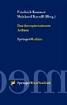Buch SFr. 12.90Buch SFr. 27.90Buch SFr. 30.90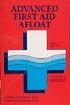Buch SFr. 69.00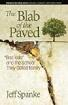Buch SFr. 21.90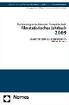Buch SFr. 37.90Buch SFr. 23.90Buch

AGB | Datenschutzerklärung | Mein Konto | Impressum | Partnerprogramm
Newsletter |Newsfeed |Facebook |Twitter